# The swallow

The swallow will fly 2.8 km per minute. How many km will the swallow fly in one hour?

x =  168 km

### Step-by-step explanation: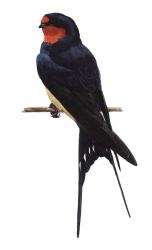Did you find an error or inaccuracy? Feel free to write us. Thank you!Tips to related online calculators
Do you want to convert length units?
Do you want to convert velocity (speed) units?
Do you want to convert time units like minutes to seconds?

## Related math problems and questions:

• Walking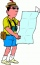The boy walked about 8.5 km in an hour. How long will it take to walk a distance of 32 km if he takes two breaks of 30 minutes during the route?
• Avg speed of flight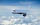The students vice adventure had a 2,367 km flight. If they travel time was 2 hours and 56 minutes, what was their average speed in kilometres per hour?
• TouristTourist walk at 4.1 km/h. Every 5 km have 20-minute break. How many kilometers passed, when started at 4:40 and finished at 10:30?
• Car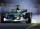Car is traveling 98 km per hour. How long-distance it travel in 1 minute?
• The car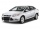The car travels at a speed of 70 km/h, what distance will it travel in 2 hours?
• Heart as pump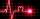Heart pumps out 5.17 liters of blood in 1 minute. How many liters of blood pumped per hour and how much per day?
• Cyclist 12What is the average speed of a cycle traveling at 20 km in 60 minutes in km/h?
• Pedestrian and cyclist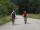Pedestrian out with a speed of 4 km/hour from city center and after 1hour and 10 minutes came after him cyclist at speed of 18 km/h. At how many minutes he catches up with pedestrian?
• Speeds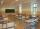George pass on the way to school distance 200 meters in 165 seconds. What is the average walking speed in m/s and km/h?
• Cyclist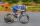A cyclist passes 88 km in 4 hours. How many kilometers did he pass in 8 hours?
• Train and walker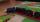Walker passes an hour 5 km. Express train per hour 100 km. Walker went 4 hours. How many minutes took the distance express train?
• Cheetah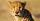Cheetah elapses 150 m for 5 seconds, the eagle reaches speeds up to 230 km/h, dolphin swims 1.2 km per minute. Which animal is the fastest and slowest? The results write as meters per second (m/s).
• Cyclist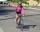Cyclist drives 5 km in half an hour, how long will drive in 3 hours?
• Perimeter of the park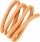Find the perimeter of the park in meters in a diamond shape, if the length of its side is 2 km.
• A plane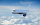A plane is flying at the rate of 350 mph after an eight hour flight is at its destination how far did the plane fly?
• Aircraft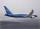If an airplane flies 846 km/h for 8 h, how far does it travel?
• Speed of carIn 2 hours 40 mins, a car travels 100km. At what speed is the car traveling?